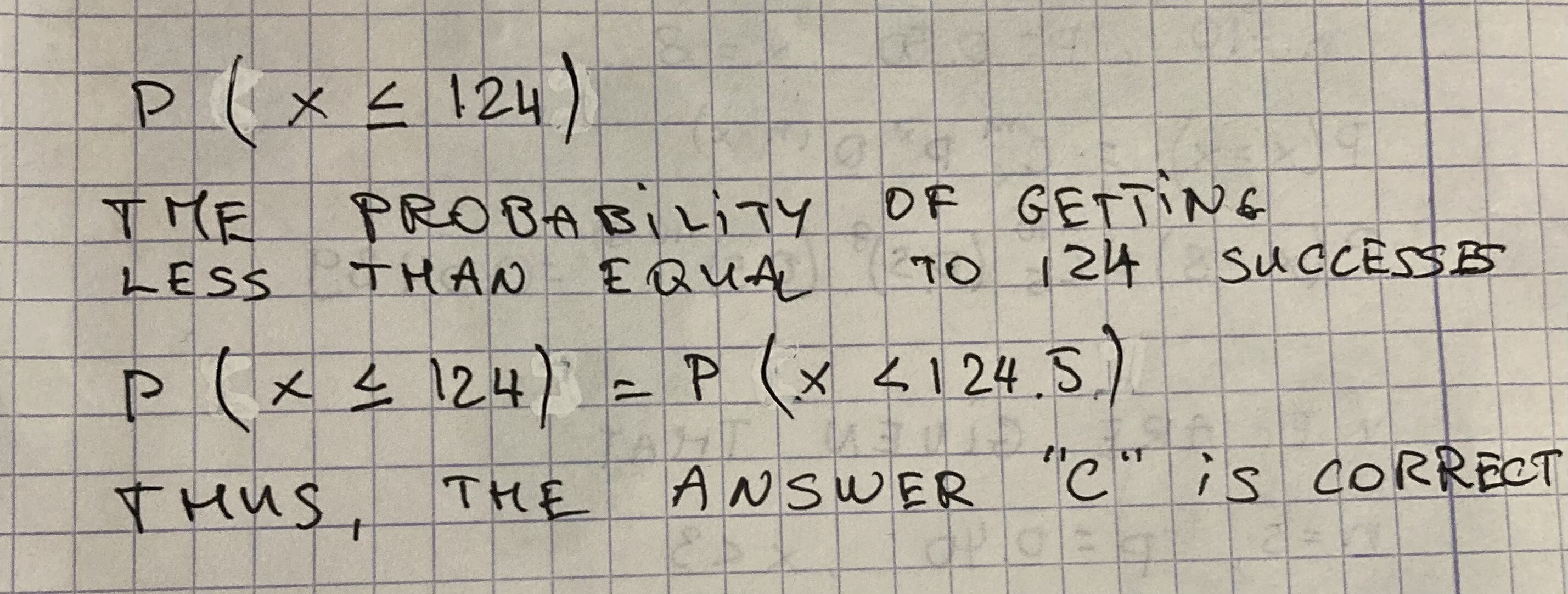Question# A binomial probability is given: P(x <= 124)Find the answer that corresponds to the binomial probability statement. a) P(x > 123.5)b) P(x < 123.5)c) P(x < 124.5)d) P(x > 124.5)e) P(123.5 < x < 124.5)

Binomial probability
ANSWEREDA binomial probability is given: $$P(x \leq 124)$$ Find the answer that corresponds to the binomial probability statement.

a) $$P(x > 123.5)$$

b) $$P(x < 123.5)$$

c) $$P(x < 124.5)$$

d) $$P(x > 124.5)$$

e) $$P(123.5 < x < 124.5)$$